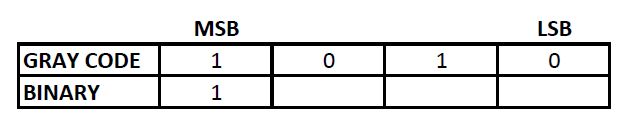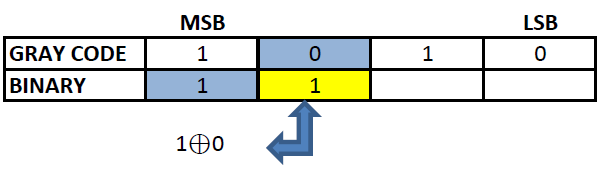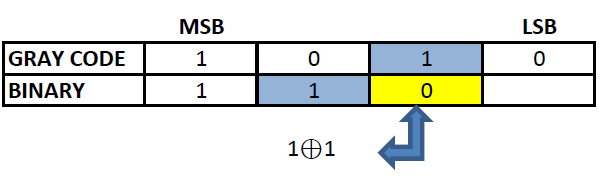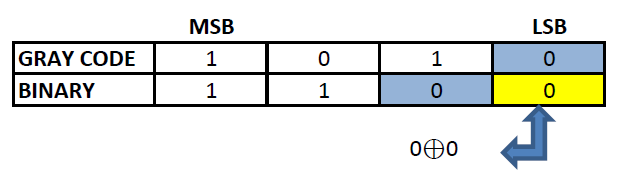Use the below tool to convert gray code to binary

 Gray Code Binary Code

Also Try:

## Binary number

Binary numbering system uses only two symbols 0 and 1. Each digits of a binary number is referred to as bits. Binary system is also known as base -2 system. Each digit is represented by the increasing power of 2 from the LSB (Least Significant Bit). Binary system is the heart of digital electronics and is used for information flow. In digital electronics, 0 and 1 are used to denote logic states, high and low. Arithmetic operations are also possible in binary system.

## Gray code

Gray code, also known as reflected binary code, is a code having digits 0 and 1. Gray code do not have place value for its digits. Any successive codes in Gray code system have only one bit changes.

## Gray code to binary conversion can be made easy with an example:

Let us convert 1010 from gray code to binary value:

Step 1: The MSB (Most Significant Bit) of a binary and gray code will be the same.Step 2: The next digit of binary will be the EXOR of the MSB of binary and the second MSB of the gray code.Step 3: Similarly EXOR the digit obtained in the previous step and the third MSB of gray code to obtain the third MSB of binary.Step 4: Repeat the previous step till the LSB of gray code is found.## Gray to Binary Conversion table

DecimalGrayBinary
000000000
100010001
200110010
300100011
401100100
501110101
601010110
701000111
810001100
911011001
1011111010
1111101011
1210101100
1310111101
1410011110
1510001111

Also Try: